Open in App
Not now

# How to Calculate Square Footage?

• Last Updated : 20 Aug, 2022

Square footage, or square feet, is a unit that is generally used to express the surface area. Square footage is used to find the measurement of the size of a room, a tile, a field, or a building for a construction project, etc. The square footage formula that is used to determine the surface area varies from one surface to the other based on the shape of the surface. The measurements of the surface are taken in terms of feet and the square footage of a surface is expressed in square feet, sq. ft., ft2. Let’s study the square footage formula in detail.

## Square Footage Formulas

For some of the geometric shapes like squares, circles, rectangles, and triangles, we can find the area or square footage with simple formulas as given below.

Take look at the square footage formulas for different geometric figures listed below:

### Square Footage Formula for a Triangle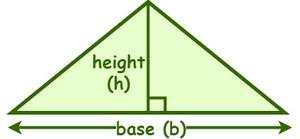If the shape is a triangle, then the square footage or the area of a triangle is calculated by finding the product of the base (b) and height (h), and then the result is multiplied by half. The formula for the square footage of a triangle is given as follows:

Area of a triangle =  ½ × b × h

where, “b” and “h” are the base and height of a triangle measured in terms of the feet.

#### Square Footage Formula for a Circle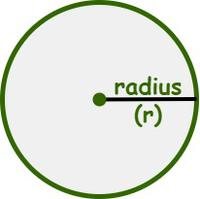To compute the square footage or area of a circle, we need the radius (r) of the circle that is measured in terms of feet. The formula for the area of a circle is given as follows:

Area of a circle = πr2

Where “r” is the radius of the circle which is measured in terms of the feet.

#### Square Footage Formula for a Parallelogram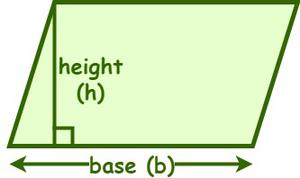If the shape is a parallelogram, then the square footage or the area of a parallelogram is calculated by finding the product of the base (b) and height (h). The formula for the area of a parallelogram is given as follows:

Area of a Parallelogram = b × h

Where “b” and “h” are the base and height of a parallelogram measured in terms of the feet.

#### Square Footage Formula for a Trapezoid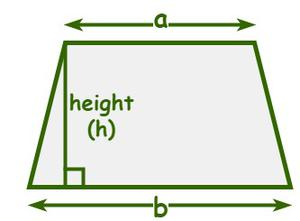To calculate the square footage or area of a trapezoid, we need the lengths of its parallel sides and the perpendicular distance between them. The formula for the area of a trapezoid is given as follows:

Area of trapezoid = ½ × h(a + b)

Where “h” is the perpendicular distance between the parallel sides,

“a” and “b” are the lengths of the parallel sides measured in terms of the feet.

#### Square Footage Formula for a Rectangle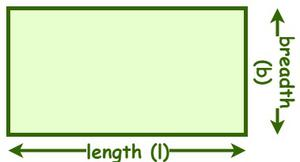If the shape is a rectangle, then the square footage or the area of a rectangle is calculated by finding the product of the length (l) and breadth (b). The formula for the area of a rectangle is given as follows:

Area of a rectangle = l × b

Where “l” and “b” are the length and breadth of a rectangle measured in terms of the feet.

#### Square Footage Formula for a Square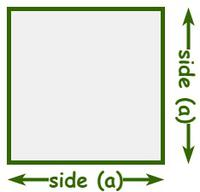If the shape is a square, then the square footage or the area of a square is calculated by finding the square of its side length since every side of the square measures the same. The formula for the area of a square is given as follows:

Area of a square = a2

Where “a” is the side length of a square measured in terms of the feet.

## Solved Examples based on Square Footage Formulas

Example 1: Calculate the total area (in square feet) of the circular field if its radius is 14 ft. [π = 22/7]

Solution:

Given,

The radius of the circular field (r) = 14 ft

By using the square footage formula of a circle,

Area of a circle = πr2

= 22/7 × (14)2

= 22/7 × 196 = 616 sq. ft

Hence, the area of the circular field is 616 sq. ft.

Example 2: Find the area or surface footage of a parallelogram whose base and height are 8 ft and 12 ft, respectively.

Solution:

Given,

The base of a parallelogram = 8 ft

The height of the parallelogram = 12 ft

By using the square footage formula of a parallelogram,

Area of a parallelogram = base × height

= 8 × 12 = 96 sq. ft

Example 3: A room is in the shape of a trapezoid. Calculate the square footage of the room if the lengths of its parallel sides are 12 ft and 15 ft, and the perpendicular distance between them is 20 ft.

Solution:

Given,

The perpendicular distance between parallel sides (h) = 20 ft.

The lengths of its parallel sides are 12 ft and 15 ft

a = 12 ft and b = 15 ft

By using the square footage formula of a trapezoid,

Area of a trapezoid = ½ × h (a + b)

= ½ × 20 × (12 + 15)

= 10 × 27 = 270 sq. ft

Hence, the square footage of the room is 270 sq. ft.

Example 4: Determine the square footage of a rectangular field if its length and breadth are 50 ft and 34 ft, respectively.

Solution:

Given,

Length (l) of a rectangular field = 50 ft

Breadth (b) of the rectangular field

By using the square footage formula of a rectangle,

Area of a rectangle = length × breadth

= 50 × 34

= 1700 sq. ft.

Hence, the square footage of a rectangular field is 1700 sq. ft.

Example 5: Calculate the height of a triangular surface whose area and base length are 280 sq. ft. and 21 ft., respectively.

Solution:

Given,

The total area of the triangular surface = 280 sq. ft

The base length of the triangular surface = 21 ft.

We have,

The area of the triangular surface = ½ × base × height

⇒ 280 = 1/2 × 21 × h

⇒ 21h = 560

⇒ h = 560/21 = 26.67 ft

Hence, the height of the triangular surface is 26.67 ft.

## FAQs on Square Footage Formulas

Question 1: Define Square Footage.

Square footage is the measurement used to calculate the area. The area of any two-dimensional shapes can be measured in square footage. To find the square footage, simply multiply the length and width.

Question 2: How big is 10000 sq. ft.

10000 sq. ft. is visualized as the square of side 100 feet. i.e. 10000 sq. ft. = 100 ft. × 100 ft.

Question 3: How many square feet are in 1 acre?

Acre is a bigger unit used for measurement of areas.1 acre contains 43560 sq. ft.

Question 4: How many square feet are in 1 square meter?